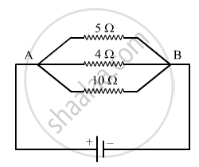Share

# In the Circuit Diagram Given Below, the Current Flowing Across 5 Ohm Resistor is 1 Amp. Find the Current Flowing Through the Other Two Resistors. - CBSE Class 10 - Science

ConceptElectric Potential Difference

#### Question

In the circuit diagram given below, the current flowing across 5 ohm resistor is 1 amp. Find the current flowing through the other two resistors.#### Solution

Given I = 1 A (Across 5 Ω)
= 5 Ω
The potential drop across AB, =IxxR
or  = 5 Ω x 1 A = 5 V
In a parallel circuit, the potential difference across the ends of all resistors remains the same. Therefore, the potential difference across 4 Ω and 10 Ω will be 5 V:
The current flowing through the 4 Ω resistor, V/R.
Here, = 5 V
= 4 Ω
So,  = 5/4 = 1.25 A
The current flowing through the 10 Ω resistor, V/R.
Here, = 5 V
R = 10 Ω
So.   = 5/10 = 0.5 A

Is there an error in this question or solution?

#### Video TutorialsVIEW ALL 

Solution In the Circuit Diagram Given Below, the Current Flowing Across 5 Ohm Resistor is 1 Amp. Find the Current Flowing Through the Other Two Resistors. Concept: Electric Potential Difference.
S